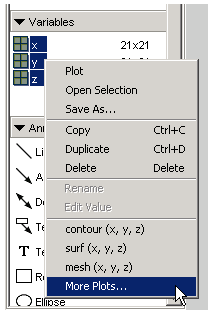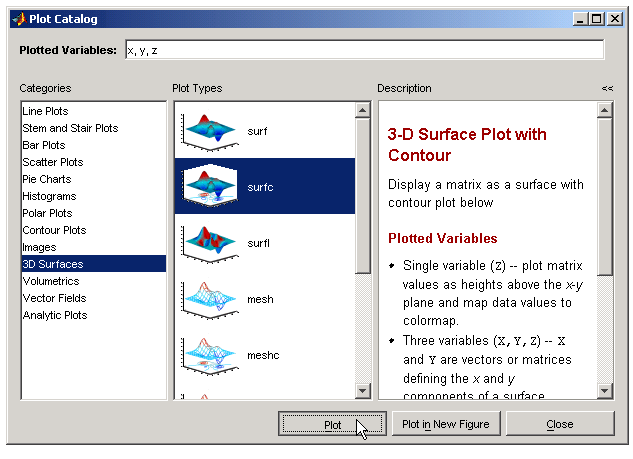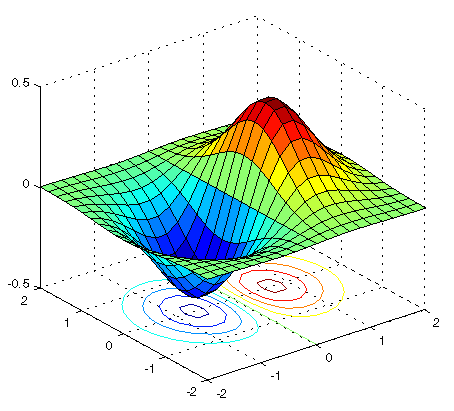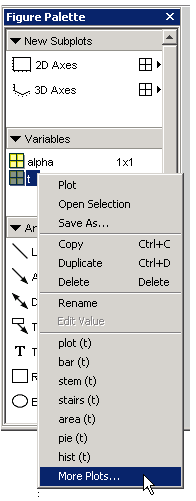GraphicsExample -- Plotting Workspace Variables

This example shows how to use the Figure Palette to select variables to plot. Suppose you have three variables in your workspace (`x`, `y`, `z`) created by the following statements:

• ```[x,y] = meshgrid([-2:.2:2]);
z = x.*exp(-x.^2-y.^2);
```

You decide to visualize this data as a surface/contour plot (as produced by the `surfc` function).

The first step is to display a figure with the Figure Palette tool attached. You can do this with the `figurepalette` command.

• ```figurepalette
```

Expand the Variables panel and shift-click (for multiple selection) on the three variables you want to pass the plotting function. Since `surfc` is not in the list, select More Plots.From the Plot Catalog tool, select the 3D Surfaces in the Categories column and surfc as the Plot Type, as shown in the following picture. To create the plot, click the Plot button.MATLAB creates the following graph.Plotting Expressions

You can enter MATLAB expressions in the Plot Catalog tool, as well as variables. For example, suppose you have created the following variables in the workspace.

• ```t = 0:.01:20;
alpha =.055;
```

and you want to plot `t` vs. this expression:

• ```exp(-alpha*t).*sin(.5*t)
```

The first step is to display a figure with the Figure Palette tool attached. You can do this with the `figurepalette` command.

• ```figurepalette
```

First select the variable `t` and right-click to display the context menu. Select More Plots.When the Plot Catalog tool is displayed, add the expression to the Plotted Variables text field. Note that you can reference the variable `alpha` because you created it in the base workspace. See MATLAB Workspace for information about variables in the MATLAB workspace.Click the Plot button to create the graph. Note that the previous step is the equivalent of issuing the following command:

• ```plot(t,exp(-alpha*t).*sin(.5*t))
```Adding a Subplot Example -- Specifying a Data Source© 1994-2005 The MathWorks, Inc.